## Title

Ohm's Law

Ohm’s Law, Resistance and Resistivity

Resistance

• Current, I - Flow of electrons around the circuit (Amps): how fast the electrons travel around
• Voltage, V - Driving force that pushes electrons (Volts): electrical pressure
• Resistance, R - Slows down the electrons (Ohms): resists the flow of the electrons

Resistance = Voltage / Current

R       =     V   /   I

Unit of resistance: Ohm, Ω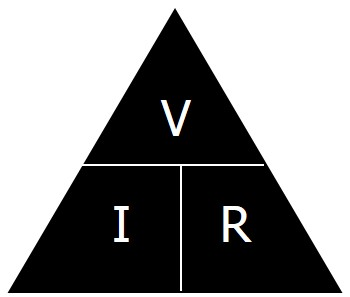The graphs below represent typical results obtained for a metal wire at constant temperature, a filament lamp, and a diode: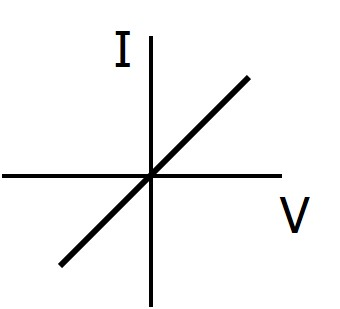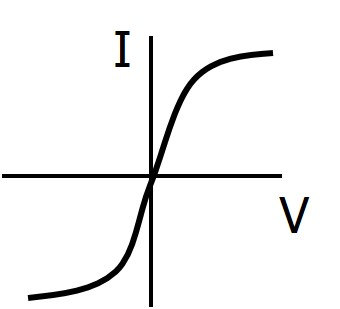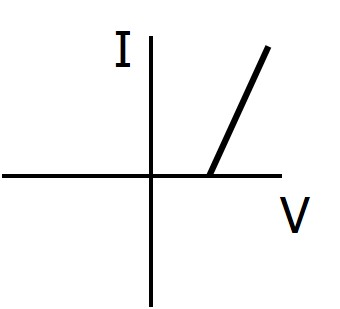Wire                                               Bulb                                            Diode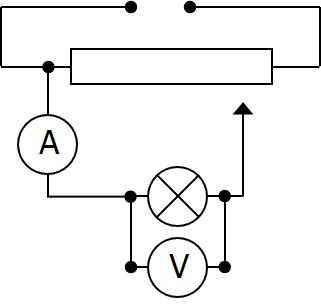A potential divider is used to investigate how the current passing in a component is dependent on the voltage across it.

Resistivity

unit: Ohm metres, Ωm

The physical dimensions and the cross sectional area have a direct effect on the resistance of a resistor. The resistance of a sample of material is directly proportional to the length and inversely proportional to its cross sectional area.

Hence:          R I / A

The resistive properties of a resistor are measured by its resistivity, ρ

When this is taken into account, the formula becomes:

R = ρL / A

where L = length of material, A = cross sectional area